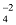# Monoatomic Ions and Polyatomic Ions

## Atom and Molecule of Class 9

Monoatomic ions : Those ions which are formed form single atoms are called monoatomic ions or simple ions.

e.g.  Na+, Mg2+ etc.

Polyatomic ion

Ions formed from a group of atoms carrying a charge (either negative or positive) are known as a polyatomic ion or compound ion.

For example.

## IONIC COMPOUNDS

Those compounds which are made up of ions (cations and anions) and are held together by strong electrostatic forces of attraction are called ionic compounds.

The forces which hold the ions together in an ionic compound are known as ionic bonds or electrovalent bonds.

e.g. Calcium nitrate Ca(NO3)2 is an ionic compound, whose one molecule is made up of one calcium ion (Ca2+) and two nitrate ions (NO3), making the overall charge on calcium nitrate zero.

Overall charge on an ionic compound is always zero

Some of the common ionic compound, their formula and the ions present in them are given below :

### Some Ionic compounds

 Name Formula Ions present 1. sodium chloride NaCl Na+ and Cl− 2. Potassium chloride KCl K+ and Cl− 3. Ammonium chloride NH4Cl NH4+ and Cl− 4. Magnesium MgCl2 Mg2+ and Cl− 5. Calcium chloride CaCl2 Ca2+ and Cl− 6. Magnesium oxide MgO Mg2+ and O2− 7. Calcium oxide CaO Ca2+ and O2− 8. Aluminium oxide Al2O3 Al3+ and O2− 9. Sodium hydroxide NaOH Na+ and OH− 10. Copper sulphate CuSO4 Cu2+ and SO11. Calcium nitrate Ca(NO2)2 Ca2+ and NO3−

As discussed earlier a compound is always made up of the same elements combined together in a fixed proportion by mass. For example Hydrogen chloride contains hydrogen and chlorine in a fixed ratio of 1 : 35.5 by mass. Similarly calcium oxide contains calcium and oxygen in the fixed ratio of 40 : 16 or 5 : 2 by mass.

### Some ionic compounds

Ionic Compound

Constituting Elements

Ratio by Mass

Calcium oxide

Magnesium

Sulphide

Sodium chloride

Calcium and oxygen

Magnesium and sulphur

Sodium and chlorine

5:2

3:4

23:35:5

Formula Unit of Ionic Compounds :

The simplest combination of ions that produces and electrically neutral unit,  is called  a formula unit of the ionic compound. Molecular formula of ionic compounds cannot be determined because they consist of large no. of ions. So, ionic compounds are represented by formula unit.

e.g. Sodium chloride is an ionic compound which consists of a large number of Na+ and Cl- ions (but they should be equal in number). So, the actual formula of sodium chloride should be (Na+)n(Cl-)n or (Na+Cl-)n, where ‘n’ is a large number. Nacl is the simplest formula of sodium chloride and thus, the formula unit of sodium chloride is NaCl.